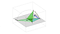Figure 1: A three-dimensional Euclidean space used to represent solutions of linear equations  . Image is a vector derivative from “High-dimensional Simplexes for Supermetric Search” by Richard Connor, Lucia Vadicamo, and Fausto Rabitti .

# Basic Linear Algebra for Deep Learning and Machine Learning Python Tutorial

## An introductory tutorial to linear algebra for machine learning (ML) and deep learning with sample code implementations in Python

Last updated, January 6, 2021

Author(s): Saniya Parveez, Roberto Iriondo

This tutorial’s code is available on Github and its full implementation as well on Google Colab.

📚 Check out our editorial recommendations on the best laptops for machine learning, data science, and deep learning. 📚

# Introduction

The foundation of machine learning and deep learning systems wholly base upon mathematics principles and concepts. It is imperative to understand the fundamental foundations of mathematical principles. During the baseline and building of the model, many mathematical concepts like the curse of dimensionality, regularization, binary, multi-class, ordinal regression, and others must be artistic in mind.

The basic unit of deep learning, commonly called a neuron, is wholly based on its mathematical concept, and such involves the sum of the multiplied values involving input and weight. Its activation functions like Sigmoid, ReLU, and others, have been built using mathematical theorems.

These are the essential mathematical areas to understand the basic concepts of machine learning and deep learning appropriately:

• Linear Algebra.
• Vector Calculus.
• Matrix Decomposition.
• Probability and Distributions.
• Analytic Geometry.

# Linear Algebra in Machine Learning and Deep Learning

Linear algebra plays a requisite role in machine learning due to vectors’ availability and several rules to handle vectors. We mostly tackle classifiers or regressor problems in machine learning, and then error minimization techniques are applied by computing from actual value to predicted value. Consequently, we use linear algebra to handle the before-mentioned sets of computations. Linear algebra handles large amounts of data, or in other words, “linear algebra is the basic mathematics of data.”

These are some of the areas in linear algebra that we use in machine learning (ML) and deep learning :

• Vector and Matrix.
• System of Linear Equations.
• Vector Space.
• Basis.

Also, these are the areas of machine learning (ML) and deep learning, where we apply linear algebra’s methods:

• Derivation of Regression Line.
• Linear Equation to predict the target value.
• Support Vector Machine Classification (SVM).
• Dimensionality Reduction.
• Mean Square Error or Loss function.
• Regularization.
• Covariance Matrix.
• Convolution.

# Matrix

A matrix is an essential part of linear algebra. It stores m*n elements of data, and we use it for the computation of the linear equation system or the linear mappings. It is an m*n tuple of real-value elements .

The number of rows and columns is called the dimension of the matrix.

# Vector

In linear algebra, a vector is an n*1 matrix. It has only one column.

# Matrix Multiplication

Matrix multiplication is a dot product of rows and columns where the row of the matrix is multiplied and summed up with another matrix column.

## In Linear Regression

There are multiple features to predict the price of a house. Below is the table of different houses with their features and the target value (price).

Therefore, to calculate the hypothesis:

# Transpose Matrix

For A ∈ R^m*n the matrix B ∈ R^n*m with bij = aij is called transpose of A. It is represented as B = A^T.

Example:

# Inverse Matrix

Consider a square matrix A ∈ R^n*n. Let matrix B∈R^n*n has the property that AB = In = BA, B is called the inverse of A and denoted by A^-1.

# Orthogonal Matrix

A square matrix A∈R^n*n is an orthogonal matrix if and only if its columns are orthonormal (unit length) so that:

Example:

Consequently,

# Diagonal Matrix

A square matrix A∈R^n*n is a diagonal matrix where all the elements are zero except those on the main diagonal like:

Aij =0 for all i != j

Aij = 0 for some or all i = j

Example:

# Transpose Matrix and Inverse Matrix in Normal Equation

The normal equation method minimizes J by explicitly taking its derivatives concerning theta j and setting them to zero. We can directly find out the value of θ without using Gradient Descent .

Implementation by taking the data from the table above, “Table1” is shown in figure 5.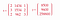Figure 19: Features under matrix x and prices under matrix y.

Create matrices of features x and target y:

`import numpy as np Featuresx = np.array([[2, 1834, 1],[3, 1534, 2],[2, 962, 3]])# Target or Pricey = [8500, 9600, 258800]`

Transpose of matrix x:

`# Transpose of xtranspose_x = x.transpose()transpose_x`

Multiplication of transposed matrix with original matrix x:

`multi_transpose_x_to_x = np.dot(transpose_x, x)multi_transpose_x_to_x`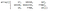Figure 21: Multiplication of the transposed matrix with the original matrix.

The inverse of the multiplication of the transposed matrix with the original matrix

`inverse_of_multi_transpose_x_to_x = np.linalg.inv(multi_transpose_x_to_x)inverse_of_multi_transpose_x_to_x`

Multiplication of transposed x with y:

`multiplication_transposed_x_y = np.dot(transpose_x, y)multiplication_transposed_x_y`

Calculation of theta’s value:

`theta = np.dot(inverse_of_multi_transpose_x_to_x, multiplication_transposed_x_y)theta`

# Linear Equation

The linear equation is the central part of linear algebra by which many problems are formulated and solved. It is an equation for a straight line.

We represent the linear equation in figure 24:

Example: x = 2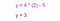Figure 27: Derivative of y from the linear equation shown above.

## Linear Equations in Linear Regression

Regression is a process that gives the equation for the straight line. It tries to find a best-fitting line with a specific set of data. The equation of the straight line bases on the linear equation:

Y = bX + a

Where,

a = It is a Y-intercept and determines the point where the line crosses the Y-axis.

b = It is a slope and determines the direction and degree to which the line is tilted.

Implementation

Predict the price of the house where the variables are square feet and price.

`import pandas as pddf = pd.read_csv('house_price.csv')df.head()`

Calculating the mean:

`def get_mean(value):    total = sum(value)    length = len(value)    mean = total/length    return mean`

Calculating the variance:

`def get_variance(value):    mean = get_mean(value)    mean_difference_square = [pow((item - mean), 2) for item in value]    variance = sum(mean_difference_square)/float(len(value)-1)    return variance`

Calculating the covariance:

`def get_covariance(value1, value2):    value1_mean = get_mean(value1)    value2_mean = get_mean(value2)    values_size = len(value1)    covariance = 0.0    for i in range(0, values_size):        covariance += (value1[i] - value1_mean) * (value2[i] - value2_mean)    return covariance / float(values_size - 1)`

Linear regression implementation:

`def linear_regression(df):    X = df['square_feet']    Y = df['price']    m = len(X)    square_feet_mean = get_mean(X)    price_mean = get_mean(Y)        #variance of X    square_feet_variance = get_variance(X)    price_variance = get_variance(Y)        covariance_of_price_and_square_feet = get_covariance(X, Y)    w1 = covariance_of_price_and_square_feet        float(square_feet_variance)    w0 = price_mean - w1 * square_feet_mean        # prediction --> Linear Equation    prediction = w0 + w1 * X        df['price (prediction)'] = prediction    return df['price (prediction)']`

Calling the ‘linear_regression’ method:

`linear_regression(df)`

The linear equation used in the method “linear_regression”:

# Vector Norms

Vector norms measure the magnitude of a vector . Fundamentally, the size of a given variable x can be represented by its norm ||x||, and the norm represents the distance between two variables x and y, and it is represented by ||x-y||.

The general equation of the vector norm:

These are the general classes of p-norms:

• L1 norm or Manhattan Norm.
• L2 norm or Euclidean Norm.

L1 and L2 norms are used in Regularization.

# L1 norm or Manhattan Norm

The L1 norm on R^n is defined for x ∈ R^n as shown in figure 31:

As shown in figure 32, the red lines symbolize the set of vectors for the L1 norm equation.

# L2 norm or Euclidean Norm

The L2 norm of x∈R^n is defined as:

As shown in figure 34, the red lines symbolize the set of vectors for the L2 norm equation.

# Regularization in Machine Learning

Regularization is a process of modifying the loss function to penalize specific values of the weight on learning. Regularization helps us avoid overfitting.

It is an excellent addition in machine learning for the operations below:

• To handle collinearity.
• To filter out noise from data.
• To prevent overfitting.
• To get good performance.

These are the standard regularization techniques:

• L1 Regularization (Lasso)
• L2 Regularization (Ridge)

Regularization is the application of the norm.

## L1 Regularization (Lasso)

Lasso is a widespread regularization technique. Its formula is shown in figure 35:

## L2 Regularization (Ridge)

The equation of L2 regularization (Ridge):

Where, λ = Controls the tradeoff of complexity by adjusting the weight of the penalty term.

# Feature Extraction and Feature Selection

The main intention of feature extraction and feature selection is to pick an optimal set of lower dimensionality features to enhance classification efficiency. These terms essentially treat the curse of the dimensionality problem. Feature selection and feature extraction are performed in the matrix.

## Feature Extraction

In feature extraction, we find a set of features from the existing features through some function mapping.

## Feature Selection

In feature selection, select a subset of the original features.

Main feature extraction methods are:

• Principal Component Analysis (PCA)
• Linear Discriminant Analysis (LDA)

PCA is a critical feature extraction method, and it is vital to know the concepts of the covariance matrix, eigenvalues, or eigenvectors to understand the concept of PCA.

# Covariance Matrix

The covariance matrix is an integral part of PCA derivation. The below concepts are important to compute a covariance matrix:

• Variance.
• Covariance.

## Variance

or

The limitation of variance is that it does not explore the relationship between variables.

## Covariance

Covariance is used to measure the joint variability of two random variables.

# Covariance Matrix

A covariance matrix is a squared matrix that gives the covariance between each pair of given random vector elements.

The equation of the covariance matrix:

# Eigenvalues and Eigenvectors

The definition of Eigenvalues:

Let m be an n*n matrix. A scalar λ is called the eigenvalue of m if there exists a non-zero vector x in R^n such that mx = λx.

and for Eigenvector:

The vector x is called an eigenvector corresponding to λ.

## Calculation of Eigenvalues & Eigenvectors

Let m be n*n matrix with eigenvalues λ and corresponding eigenvector x. So, mx = λx. This equation can be written as below:

mx — λx = 0

So, the equation: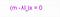Figure 45: Solving this equation for λ gives us all the eigenvalues of m.

Example:

Calculate eigenvalues and eigenvectors of given matrix m:

Solution:

Here, the size of the matrix is 2. So:

Here, the Eigenvalues of m are 2 and -1.

There are multiple eigenvectors available to each eigenvalue.

# Orthogonality

Two vectors v and w are called orthogonal if their dot product is zero .

v.w = 0

Example:

# Orthonormal Set

If all vectors in the set are mutually orthogonal and all of the unit lengths, then it is called an Orthonormal set . An orthonormal set that forms a basis is called an orthonormal basis.

# Span

Let V is a vector space and its elements are v1, v2, ….., vn ∈ V.

Any sum of these elements multiplied by the scalars representing the equation shown in figure 53, a set of all linear combinations is called the span.

Example:

Hence:

Span (v1, v2, v3) = av1 + bv2 + cv3

# Basis

A basis for a vector space is a sequence of vectors that form a set that is linearly independent and that spans the space .

Example:

The vector’s sequence below is a basis:

It is linearly independent as given below:

# Principal Component Analysis (PCA)

PCA endeavors a projection that processes as much knowledge in the data as reasonable. It is a dimensionality reduction technique. It finds the directions of the highest variance and projects the data with them to decrease the dimensions.

Calculation steps of PCA:

Let there is an N*1 vector with values x1, x2, ….., xm.

• Calculate the sample mean:
• Subtract sample mean with vector value:
• Calculate the sample covariance matrix:
• Calculate the eigenvalues and eigenvectors of the covariance matrix
• Dimensionality reduction: approximate x using only the first k eigenvectors (k< N).

## Python Implementation of the Principal Component Analysis (PCA)

The main goal of Python’s implementation of the PCA:

• Implement the covariance matrix.
• Derive eigenvalues and eigenvectors.
• Understand the concept of dimensionality reduction from the PCA.

`import numpy as npimport pylab as plimport pandas as pdfrom sklearn import datasetsimport matplotlib.pyplot as pltfrom sklearn.preprocessing import StandardScalerload_iris = datasets.load_iris()iris_df = pd.DataFrame(load_iris.data, columns=[load_iris.feature_names])iris_df.head()`

Standardization

It is always good to standardize the data to keep all features of the data in the same scale.

`standardized_x = StandardScaler().fit_transform(load_iris.data)standardized_x[:2]`

Compute Covariance Matrix

`covariance_matrix_x = np.cov(standardized_x.T)covariance_matrix_x`

Compute Eigenvalues and Eigenvectors from Covariance Matrix

`eigenvalues, eigenvectors = np.linalg.eig(covariance_matrix_x)eigenvalues`
`eigenvectors`

Check variance in Eigenvalues

`total_of_eigenvalues = sum(eigenvalues)varariance = [(i / total_of_eigenvalues)*100 for i in sorted(eigenvalues, reverse=True)]varariance`

The values shown in figure 68 indicate the variance giving the analysis below:

• 1st Component = 72.96%
• 2nd Component = 22.85%
• 3rd Component = 3.5%
• 4th Component = 0.5%

So, the third and fourth Components have very low variance respectively. These can be dropped. Because these components can’t add any value.

Taking 1st and 2nd Components only and Reshaping

`eigenpairs = [(np.abs(eigenvalues[i]), eigenvectors[:,i]) for i in range(len(eigenvalues))]# Sorting from Higher values to lower valueeigenpairs.sort(key=lambda x: x, reverse=True)eigenpairs`

Perform Matrix Weighing of Eigenparis

`matrix_weighing = np.hstack((eigenpairs.reshape(4,1),eigenpairs.reshape(4,1)))matrix_weighing`

Multiply the standardized matrix with matrix weighing:

`Y = standardized_x.dot(matrix_weighing)Y`

Plotting

`plt.figure()target_names = load_iris.target_namesy = load_iris.targetfor c, i, target_name in zip("rgb", [0, 1, 2], target_names):    plt.scatter(Y[y==i,0], Y[y==i,1], c=c, label=target_name)plt.xlabel('PCA 1')plt.ylabel('PCA 2')plt.legend()plt.title('PCA')plt.show()`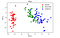Figure 73: The plotted result of the PCA 1 and PCA 2 from the first dataset.

# Matrix Decomposition or Matrix Factorization

Matrix Decomposition or factorization is also an important part of linear algebra used in machine learning. Basically, it is a factorization of the matrix into a product of matrices.

There are several techniques of matrix decomposition like LU decomposition, Singular value decomposition (SVD), etc.

Singular Value Decomposition (SVD)

It is a technique for the reduction of dimension. As per singular value decomposition matrix:

Let M is a rectangular matrix and can be broken down into three products of matrix — (1) orthogonal matrix (U), (2) diagonal matrix (S), and (3) transpose of the orthogonal matrix (V).

# Conclusion

Machine learning and deep learning has been build upon the concept of mathematics. A vast area of mathematics is used to build algorithms and also for the computation of data.

Linear algebra is the study of vectors  and several rules to manipulate vectors. It is a key infrastructure, and it covers many areas of machine learning like linear regression, one-hot encoding in the categorical variable, PCA (Principle component analysis) for the dimensionality reduction, matrix factorization for recommender systems.

Deep learning is completely based on linear algebra and calculus. It is also used in several optimization techniques like gradient descent, stochastic gradient descent, and others.

Matrices are an essential part of linear algebra that we use to compactly represent systems of linear equations, linear mapping, and others. Also, vectors are unique objects that can be added together and multiplied by scalars that produce another object of similar kinds. Any suggestions or feedback is crucial to continue to improve. Please let us know in the comments if you have any.

DISCLAIMER: The views expressed in this article are those of the author(s) and do not represent the views of Carnegie Mellon University, nor other companies (directly or indirectly) associated with the author(s). These writings do not intend to be final products, yet rather a reflection of current thinking, along with being a catalyst for discussion and improvement.

Published via Towards AI

# Resources:

Linear Algebra, Dive Into Deep Learning, https://d2l.ai/chapter_preliminaries/linear-algebra.html

# References

 Linear Algebra, Wikipedia, https://en.wikipedia.org/wiki/Linear_algebra

 Euclidean Space, Wikipedia, https://en.wikipedia.org/wiki/Euclidean_space

 High-dimensional Simplexes for Supermetric Search, Richard Connor, Lucia Vadicamo, Fausto Rabitti, ResearchGate, https://www.researchgate.net/publication/318720793_High-Dimensional_Simplexes_for_Supermetric_Search

 ML | Normal Equation in Linear Regression, GeeksforGeeks, https://www.geeksforgeeks.org/ml-normal-equation-in-linear-regression/

 Vector Norms by Roger Crawfis, CSE541 — Department of Computer Science, Stony Brook University, https://www.slideserve.com/jaimie/vector-norms

 Variance Estimation Simulation, Online Stat Book by Rice University, http://onlinestatbook.com/2/summarizing_distributions/variance_est.html

 Lecture 17: Orthogonality, Oliver Knill, Harvard University, http://people.math.harvard.edu/~knill/teaching/math19b_2011/handouts/math19b_2011.pdf

 Orthonormality, Wikipedia, https://en.wikipedia.org/wiki/Orthonormality

 Linear Algebra/Basis, Wikibooks, https://en.wikibooks.org/wiki/Linear_Algebra/Basis

 Linear Algebra, LibreTexts, https://math.libretexts.org/Bookshelves/Linear_Algebra

 Linear Algebra, Dive Into Deep Learning, Aston Zhang, Zack C. Lipton, Mu Li, Alex J. Smola, https://d2l.ai/chapter_preliminaries/linear-algebra.html

Written by

Written by

## Towards AI Team

#### Publishing the Best of Tech, Science, and Engineering | Editorial → https://towardsai.net/editorial | Subscribe→ https://towardsai.net/subscribe — @Towards_AI